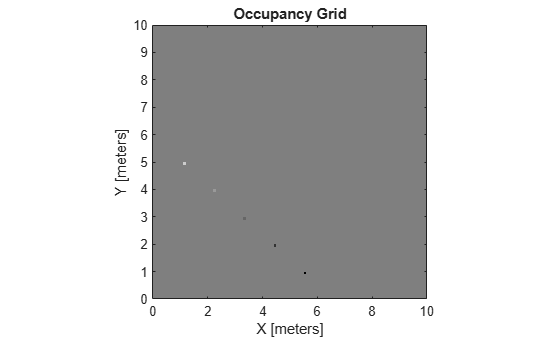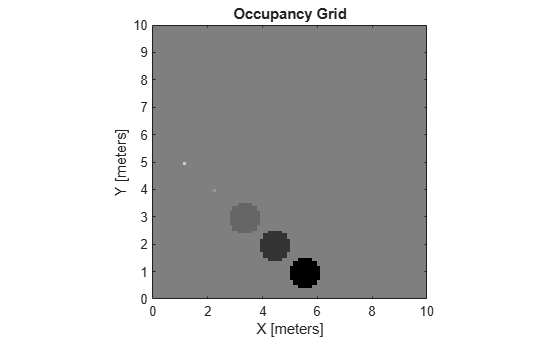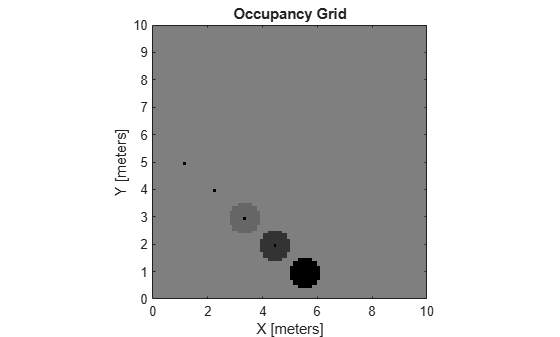# world2grid

Convert world coordinates to grid indices

## Syntax

``ij = world2grid(map,xy)``

## Description

example

````ij = world2grid(map,xy)` converts an array of world coordinates, `xy`, to an array of grid indices, `ij` in ```[row col]``` format.```

## Examples

collapse all

Create an empty map of 10-by-10 meters in size.

`map = occupancyMap(10,10,10);`

Update the occupancy of specific world locations with new probability values and display the map.

```x = [1.2; 2.3; 3.4; 4.5; 5.6]; y = [5.0; 4.0; 3.0; 2.0; 1.0]; pvalues = [0.2; 0.4; 0.6; 0.8; 1]; updateOccupancy(map,[x y],pvalues) figure show(map)```Inflate the occupied areas by a radius of 0.5 m. The larger occupancy values overwrite the smaller values.

```inflate(map,0.5) figure show(map)```Get the grid locations from the world locations.

`ij = world2grid(map,[x y]);`

Set occupancy values for the grid locations.

```setOccupancy(map,ij,ones(5,1),'grid') figure show(map)```## Input Arguments

collapse all

Map representation, specified as a `occupancyMap`, `mapLayer`, or `multiLayerMap` object.

World coordinates, specified as an n-by-2 matrix of ```[x y]``` pairs, where n is the number of world coordinates.

Data Types: `double`

## Output Arguments

collapse all

Grid indices, returned as an n-by-2 matrix of ```[i j]``` pairs in ```[row col]``` format, where n is the number of grid positions. The grid cell locations are counted from the top left corner of the grid.

Data Types: `double`